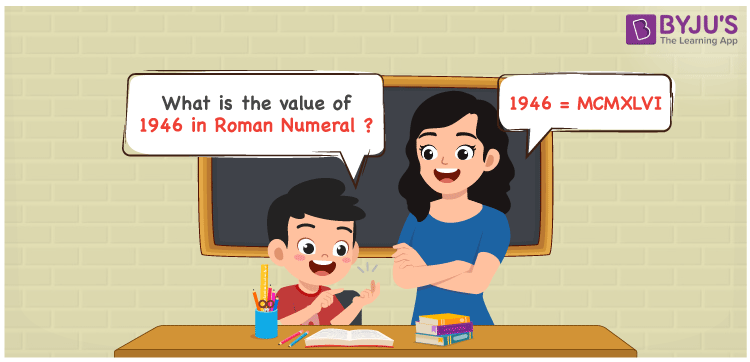# 1946 in Roman Numerals

1946 in Roman Numerals is MCMXLVI. Any Roman numeral uses only the 7 primary symbols which are I, V, X, L, C, D, and M, Where M represents 1000, C represents 100, L represents 50, X represents 10 and I represents 1. MCMXLVI represents the number 1946 which is obtained by the addition and subtraction rules of Roman Numerals. What are Roman numerals? can be referred for more details.

 Number Roman Numeral 1946 MCMXLVI

## How to Write 1946 in Roman Numerals?To convert 1946 in Roman Numerals, we need to represent 1946 as the sum of the fundamental symbols. 1946 can be broken down as

1. The number 1000,
2. Difference of 1000 and 100 to get 900
3. Difference of 50 and 10 to get 40
4. Sum of 5 and 1 to get 6
5. Add all the numbers from previous steps.

Let us understand it with Numerical representation.

1946 = 1000 + 900 + 40 + 6

1946 = 1000 + (1000 – 100) + (50 – 10) + ( 5 + 1)

1946 = M + (M – C) + (L – X) + (V + I)

1946 = M + (CM) + XL + VI

1946 = MCMXLVI.

## Video Lesson on Roman Numerals## Frequently Asked Questions on 1946 in Roman Numerals

Q1

### How to write the number 1946 in Roman Numerals?

1946 in Roman Numerals is written as MCMXLVI.
Q2

### Does MCMXLVI represent Roman Numerals?

Yes. MCMXLVI represents Roman Numerals and it is the number 1946
Q3

### What is the year 1946 in Roman numerals?

The year 1946 in Roman Numerals is MCMXLVI.